# JP6016167B2 - Printed matter, printed matter production method and production system - Google Patents

## Info

Publication number
JP6016167B2
JP6016167B2 JP2013244460A JP2013244460A JP6016167B2 JP 6016167 B2 JP6016167 B2 JP 6016167B2 JP 2013244460 A JP2013244460 A JP 2013244460A JP 2013244460 A JP2013244460 A JP 2013244460A JP 6016167 B2 JP6016167 B2 JP 6016167B2
Authority
JP
Japan
Prior art keywords
element
unit
formed
image
invisible image
Prior art date
Legal status (The legal status is an assumption and is not a legal conclusion. Google has not performed a legal analysis and makes no representation as to the accuracy of the status listed.)
Active
Application number
JP2013244460A
Other languages
Japanese (ja)
Other versions
JP2015101040A (en
Inventor

Original Assignee

Priority date (The priority date is an assumption and is not a legal conclusion. Google has not performed a legal analysis and makes no representation as to the accuracy of the date listed.)
Filing date
Publication date
Application filed by 独立行政法人 国立印刷局, 独立行政法人 国立印刷局 filed Critical 独立行政法人 国立印刷局
Priority to JP2013244460A priority Critical patent/JP6016167B2/en
Publication of JP2015101040A publication Critical patent/JP2015101040A/en
Application granted granted Critical
Publication of JP6016167B2 publication Critical patent/JP6016167B2/en
Application status is Active legal-status Critical
Anticipated expiration legal-status Critical

## Images

••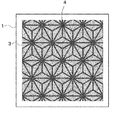•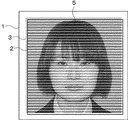•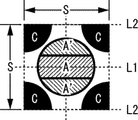•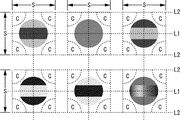•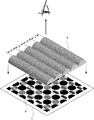•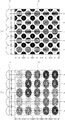•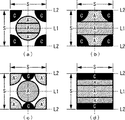•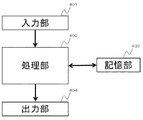••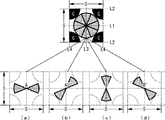•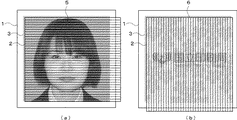•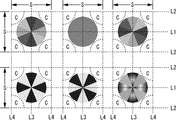•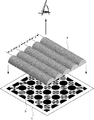•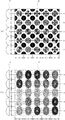•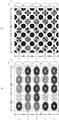••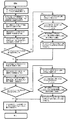•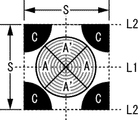•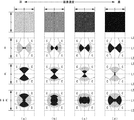•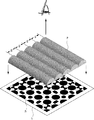••••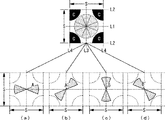••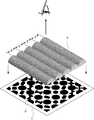•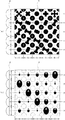•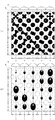•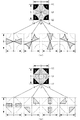•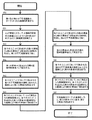•## Description

The present invention provides banknotes, stock certificates, securities such as receivables, various certificates and valuable documents, printed matter that needs to be prevented from forgery or duplication, printed matter utilized for artistic appreciation, The present invention relates to a manufacturing method and a manufacturing system.

In general, a variety of technologies are applied to valuable printed matter such as certificates in order to provide an anti-counterfeiting effect. However, in recent years, with the increase in image quality of color copiers and computerization of color platemaking technologies, The forgery technology tends to diversify. As a method for preventing these counterfeiting, there are many anti-counterfeiting techniques using special materials, which are not usually observed under light, but are provided with an invisible image observed under specific conditions.

Anti-counterfeiting technology using these special materials is visible as a uniform color under visible light, but is printed using infrared transmission / absorption pair inks that are observed in different colors under infrared light. A typical example is a printed material using a light-emitting material that is transparent and develops color when irradiated with energy rays such as ultraviolet rays, thereby causing an invisible image to appear.

The technology using printed materials and luminescent materials using infrared transmission and absorption pair inks requires special materials and introduces special equipment consisting of special machinery and equipment to obtain an environment for confirming anti-counterfeiting effects. For example, the authenticity determination means is expensive.

Therefore, the present applicant regularly forms a plurality of units that can form equal colors and lines of the same area, which are formed using general color materials, without using special materials. In each unit, the image line is arranged in one of a pair of image lines arranged so as to oppose the center to the boundary. An application has been filed for a printed matter in which an invisible image appears as a visible image when observed in a superimposed manner (for example, see Patent Document 1).

The printed matter of Patent Document 1 can arrange an image line that forms a visible image and an image line that forms a second invisible image in each regularly formed unit. A visible image is observed when observed below, and a first invisible image is observed when the discriminator is overlapped along the first direction, and the discriminator is overlapped along a second direction different from the first direction. The printed matter has an image switching effect such that the second invisible image can be observed when observed.

In addition, as in the invention of Patent Document 1, the applicant of the present invention forms a plurality of units having a line area for forming a visible image and an image area for forming at least one invisible image. Filed an anti-counterfeit printed matter that has the same effect as document 1 and has a visible image having a continuous tone that reproduces shadows from highlights by means of halftone image lines. (For example, refer to Patent Document 2).

The printed matter of Patent Document 2 arranges an image line that forms a visible image based on image data having continuous gradation such as a face photograph, and further arranges an image line that forms at least one invisible image in each unit. By doing so, an invisible image can be formed in the region where the face photograph is formed.

Further, as a printed matter in which a continuous tone is added to an invisible image that is visualized when the discriminating tool is overlaid, each line element is formed by partially changing the phase shift amount according to the density of the invisible image. Thus, a printed matter on which an invisible image having at least one continuous tone is formed is disclosed (for example, see Patent Document 3).

Japanese Patent No. 4635160 Japanese Patent No. 4915883 Japanese Patent No. 4132122

However, in the printed matter of Patent Document 1 and Patent Document 2, a pair of image lines that form an invisible image is formed with image lines of the same color and the same area, and in each unit, either one of the image lines is formed. Since the invisible image is formed by forming the image line by the negative / positive relationship of selecting and arranging, the density of the image line forming the pair of invisible images cannot be partially changed. Therefore, an invisible image having continuous gradation cannot be formed.

In addition, although the invention of Patent Document 3 can form an invisible image having a continuous tone, it is an invention that only forms an image line that forms an invisible image by an arbitrary shift amount. It does not have the effect of switching to an invisible image, is limited by the design of the printed material, and only the image line forming the invisible image is arranged, so that the image line structure can be easily decoded. There was a problem of fear.

The present invention has been considered in view of the above-described problems. The image data that forms the basis of the invisible image is cut out by the elements forming the image portion of the invisible image, and the image data obtained by negatively inverting the invisible image is used as the background of the invisible image. The invisible image having a continuous tone can be given by using a limited space in each unit by cutting out with the elements forming the part and forming each element as a pair of elements in each unit. In addition, the present invention relates to a printed matter that can provide an invisible image having continuous gradation such as a visible image and a photographic image by arranging an element that forms a visible image in each unit, and a manufacturing method thereof.

The printed matter of the present invention has a printing region in which units having the first element, the second element, and the third element are regularly formed in a plurality of matrix shapes at a predetermined pitch on at least a part of the substrate. The first element is formed on the same line along the first direction, and the second element and the third element are formed at positions shifted from the same line along the first direction. Each element has a gradation, and each second element has a gradation obtained by inverting the gradation of the first element in the same unit, and the first element forms a first invisible image. And a negative image of the first invisible image is formed by the second element, and a visible image is formed by the third element.

The gradation formed on the first element in the printed material of the present invention is formed by at least one method selected from a color image, the size of the element, the density of the element, or the density of the element. It is printed matter.

The third element in the printed matter of the present invention is a printed matter characterized in that a visible image having a continuous tone is formed by one method selected from the size of the element, the density of the element, or the density of the element. is there.

Moreover, the printed matter of the present invention is a printed matter characterized in that the length of the long side of the unit is 1 mm or less.

The unit of the printed matter of the present invention further includes a fourth element and a fifth element. The fourth element is formed on the same line along the second direction orthogonal to the first direction. Are formed at positions shifted from the same line along the first direction and from the same line along the second direction, each fourth element has a gradation, and each fifth element is The second element is formed by inverting the gradation formed on the fourth element in the same unit, the second element forms a second invisible image, and the fifth element forms a negative of the second invisible image. A printed matter characterized in that an image is formed.

The gradation formed on the fourth element in the printed matter of the present invention is formed by at least one method selected from a color image, the size of the element, the density of the element, or the density of the element. It is printed matter.

In addition, the printed matter of the present invention further includes a determination area that is superimposed on the print area by folding the base material, and the determination area includes a plurality of units formed in the print area on the transparent base material. The printed matter is characterized in that a filter or lenticular lens in which a line having the same pitch as the pitch is formed is formed on a substrate.

Further, the booklet-type printed matter having a printing region of the present invention, the booklet-type printed matter has a discrimination page in which a discrimination region is formed on a page adjacent to a page in which the print region is formed, The discrimination page is formed in a position that overlaps the print area when the discrimination page is overlapped on the page on which the print area is formed, and the discrimination area is formed in the print area on the transparent substrate. The booklet-type printed matter is characterized in that a line filter or a lenticular lens in which lines having the same pitch as a plurality of units are formed is arranged.

In addition, in the method for producing a printed matter according to the present invention, a step of inputting visible image data that is a basis of a visible image and first invisible image data that is a basis of a first invisible image, a first element, a first element, Arranging a unit of minimum unit including the second element and the third element regularly in a matrix at a predetermined pitch, producing a negative image obtained by negatively inverting the first invisible image data, and unit And the first invisible image data are combined, the first invisible image data is cut out by the first element arranged in the unit, the negative image of the unit and the first invisible image is combined, and the unit Hollowing out the negative image of the first invisible image with the arranged second element; hollowing out the visible image data with the third element; 1 element, a second element and printed matter manufacturing method characterized by comprising the step of outputting the plurality of units to a substrate having a third element.

The method for producing a printed material according to the present invention further includes a step of correcting the color of the second element so that the colors of the first element and the second element are equal in each unit. The step of correcting the color of each element of the unit confirms whether the color obtained by adding the colors of the first element and the second element formed in each unit is visually recognized as a uniform color in all the units. A step of deleting the second element arranged in the unit in which the sum of the colors of the first element and the second element is not equal in each unit, and the first formed in each unit Subtracting the RGB value of the first element formed in each unit from the RGB values that can be formed in the area of the first element, and calculating the total value of the RGB values of the elements of Each value divided by the area A manufacturing method of a printed material, characterized by a step of concentration of placing the element.

In addition, the printed matter manufacturing method of the present invention includes a visible image data that is a basis of a visible image, a first invisible image data that is a basis of a first invisible image, and a second invisible that is a basis of a second invisible image. A step of inputting image data, and a unit of a minimum unit including the first element, the second element, the third element, the fourth element, and the fifth element are regularly arranged in a matrix at a predetermined pitch. A step of producing a negative image obtained by negatively inverting the first invisible image data, a step of producing a negative image obtained by inverting the second invisible image data, and the unit and the first invisible image data. The first invisible image data by the first element arranged in the unit, and the unit and the negative image of the first invisible image are synthesized and arranged in the unit. A step of hollowing out a negative image of the first invisible image by two elements, a unit and second invisible image data are synthesized, and a second invisible image data is hollowed out by a fourth element arranged in the unit Combining the negative image of the unit and the second invisible image, hollowing out the negative image of the second invisible image by the fifth element arranged in the unit, and converting the visible image data to the third A plurality of units having a first element, a second element, a third element, a fourth element, and a fifth element are output to a substrate. This is a feature of a printed material production method.

In addition, in the printed matter manufacturing method of the present invention, the color obtained by adding the first element and the second element arranged in each unit and the color obtained by adding the fourth element and the fifth element are all units. In order to make the same color in the first element, the method further includes the step of correcting the color of the second element and / or the fifth element. A step of confirming whether or not a color obtained by adding the colors of the element and the second element is visually recognized as an equal color in all the units, and adding the first element and the second element in each unit Deleting a second element arranged in a unit whose color is not equal, calculating a total value of RGB values of the first element formed in each unit, and an area of the first element RGB that can be formed inside Subtracting the RGB value of the first element formed in each unit from the value and dividing the value by the area of the second element to obtain the density to be arranged in each second element, and the color of the fifth element Confirming whether or not the color obtained by adding the colors of the fourth element and the fifth element formed in each unit is visually recognized as a uniform color in all the units, In the unit, the step of deleting the fifth element arranged in the unit in which the color obtained by adding the fourth element and the fifth element is not equal, and the RGB value of the fourth element formed in each unit Subtracting the RGB value of the fourth element formed in each unit from the RGB value that can be formed in the area of the fourth element and dividing by the area of the fifth element For each fifth element A manufacturing method of the printed matter characterized in that it comprises a step of placement concentrations.

The printed matter producing method of the present invention includes a step of inputting visible image data that is a basis of a visible image and first invisible image data that is a basis of a first invisible image, a first element, a second element A step of regularly arranging a unit of a minimum unit including an element and a third element in a matrix form at a predetermined pitch; and a step of converting the resolution of the first invisible image data into image data in accordance with the unit pitch. , Calculating the maximum area that can be formed in the first element, combining the first invisible image data converted in resolution and the unit, and each unit corresponding to the density of the first invisible image data corresponding to each unit, A step of calculating an area formed in the first element, and an area obtained by subtracting an area of the first element formed in each unit from a maximum area that can be formed in the first element as the second element A step of hollowing out the visible image data with a third element, and a step of outputting a plurality of units having the first element, the second element, and the third element to the substrate. This is a method for producing a printed matter.

In addition, the printed matter manufacturing method of the present invention includes a visible image data that is a basis of a visible image, a first invisible image data that is a basis of a first invisible image, and a second invisible that is a basis of a second invisible image. A step of inputting image data, and a unit of a minimum unit including the first element, the second element, the third element, the fourth element, and the fifth element are regularly arranged in a matrix at a predetermined pitch. A step of converting the resolution to image data in which the first invisible image data is matched to the pitch of the unit, a step of calculating a maximum area that can be formed in the first element, and a first in which the resolution is converted Combining the invisible image data and the unit, calculating the area formed in the first element based on the density of the first invisible image data corresponding to each unit, and the unit formed in each unit Calculating the area obtained by subtracting the area of one element from the maximum area that can be formed in the first element as a second element, and converting the resolution of the second invisible image data to image data that matches the unit pitch A step of calculating a maximum area that can be formed in the fourth element, and combining the second invisible image data whose resolution has been converted and the unit, and the second invisible image data corresponding to each unit. The step of calculating the area formed in the fourth element by the concentration, and the area obtained by subtracting the area of the fourth element formed in each unit from the maximum area that can be formed in the fourth element is the fifth element. A step of calculating as follows, a step of hollowing out the visible image data by a third element, and a first element, a second element, a third element, a fourth element, and a fifth element Is a manufacturing method of a printed matter, characterized in that it comprises the step of outputting the number of units to the substrate.

The printed matter production system of the present invention includes an image input unit that inputs visible image data that is a basis of a visible image and first invisible image data that is a basis of the first invisible image, a first element, and a first element. Unit creating means for creating a plurality of units in which units of the minimum unit including the second element and the third element are regularly arranged in a matrix at a predetermined pitch; and a first invisible image input by the image input means A negative image producing means for producing a negative image obtained by reversing the negative of the data, a unit that combines the unit and the first invisible image data, and performs a hollowing process on the first invisible image data by the first element arranged in the unit. The image hollowing means, the unit and the negative image of the first invisible image are combined, and the negative image of the first invisible image is cut out by the second element arranged in the unit. A negative image hollowing means, a visible image hollowing means for hollowing out the visible image data input by the image input means by a third element, and a unit having a first element, a second element, and a third element It is a production system of printed matter characterized by having a printing means which prints on a substrate.

The printed matter production system of the present invention further includes color correction means for correcting the color of the second element in order to make the colors of the first element and the second element equal in each unit. The color correcting means for correcting the color of the second element is whether or not the color obtained by adding the colors of the first element and the second element formed in each unit is visually recognized as the same color in all the units. And a second element deletion unit that deletes the second element arranged in the unit determined by the determination unit that the color obtained by adding the first element and the second element is not equal. First element RGB value calculation means for calculating the total value of the RGB values of the first element formed in each unit, and the first element from the RGB values that can be formed in the area of the first element Each unit calculated by the RGB value calculation means And a second element correction value calculating means for subtracting the RGB value of the first element and dividing the area by the area of the second element to obtain a density to be arranged in each second element. This is a printed material production system.

The printed matter production system according to the present invention includes a visible image data that is a basis of a visible image, a first invisible image data that is a basis of a first invisible image, and a second invisible that is a basis of a second invisible image. Image input means for inputting image data, and a unit of a minimum unit including the first element, the second element, the third element, the fourth element, and the fifth element regularly in a matrix at a predetermined pitch A unit producing means for creating a plurality of units arranged in a negative image, a negative image obtained by negatively inverting the first invisible image data input by the image input means, and a negative image obtained by negatively inverting the second invisible image data. The first invisible image data is synthesized by combining the first invisible image data with the negative image producing means to be produced, the unit and the first invisible image data. A negative image of the first invisible image is formed by synthesizing the negative image of the first invisible image with the image cutout means, and the second element arranged in the unit cuts out the negative image of the first invisible image. Means, a unit and the second invisible image data, and a second invisible image positive image hollowing means for hollowing out the second invisible image data by a fourth element arranged in the unit; A negative image of the second invisible image is synthesized by combining the negative images of the two invisible images and the negative image of the second invisible image is cut out by the fifth element arranged in the unit; and input by the image input means Visible image hollowing means for hollowing out the processed visible image data by a third element, a first element, a second element, a third element, Printed matter manufacturing system characterized by having a printing means for printing a plurality of units to a substrate having a fourth element and a fifth element.

In the printed material production system of the present invention, a color obtained by adding up the first element and the second element arranged in each unit and a color obtained by adding up the fourth element and the fifth element are all displayed. In order to make the colors uniform in the unit, it further includes color correction means for correcting the color of the second element and / or the fifth element, and is formed in each unit as color correction means for correcting the color of the second element. Determining whether or not the color obtained by adding the colors of the first element and the second element is visually recognized as the same color in all the units, and the fourth element and the fifth element formed in each unit The determination means for determining whether or not the color obtained by adding the colors of the elements is visually recognized as the same color in all units, and the color obtained by adding the first element and the second element by the determination means is not equal. Remove the second element placed in the determined unit A second element deleting unit that deletes, and a fifth element that deletes the fifth element arranged in the unit determined by the determining unit that the color obtained by adding the fourth element and the fifth element is not equal. A first element RGB value calculating means for calculating the total value of the RGB values of the first element formed in each unit, and a first RGB value that can be formed in the area of the first element. The value obtained by subtracting the RGB value of the first element in each unit calculated by the element RGB value calculating means and dividing the area by the area of the second element is used as the density to be arranged in each second element. Element correction value calculation means, fourth element RGB value calculation means for calculating the total value of the RGB values of the fourth element formed in each unit, and RGB values that can be formed in the area of the fourth element To the fourth element RGB value calculation means And fifth element correction value calculation means for subtracting the RGB value of the fourth element in each unit and dividing the value by the area of the fifth element to obtain the density to be arranged in each fifth element. Is a production system for printed matter.

The printed matter production system of the present invention includes an image input unit that inputs visible image data that is a basis of a visible image and first invisible image data that is a basis of the first invisible image, a first element, and a first element. Unit creating means for creating a plurality of units in which units of the minimum unit including the second element and the third element are regularly arranged in a matrix at a predetermined pitch; and a first invisible image input by the image input means A first invisible image resolution converting means for converting the resolution of the data into image data in accordance with the unit pitch, and a first area for calculating a maximum area that can be formed in the first element as a reference area value of the first element; The invisible image data converted by the element reference area calculating means and the first invisible image resolution converting means are combined with the unit, and the first invisible image corresponding to each unit is synthesized. The first element area calculation means for calculating the area to be formed in the first element according to the density of the data, and the area of the first element for each unit calculated by the first element area calculation means, A second element area calculating unit that calculates an area subtracted from the reference area value of the first element as a second area, and a visible image data that is cut out by the third element from the visible image data input by the image input unit; A printed material production system comprising image hollowing means and printing means for printing a plurality of units having a first element, a second element, and a third element on a substrate.

Furthermore, the method for producing a printed material according to the present invention includes a visible image data that is a basis of a visible image, a first invisible image data that is a basis of a first invisible image, and a second invisible that is a basis of a second invisible image. Image input means for inputting image data, and a unit of a minimum unit including the first element, the second element, the third element, the fourth element, and the fifth element regularly in a matrix at a predetermined pitch Unit creating means for creating a plurality of units arranged in the first and invisible image resolution converting means for converting the resolution of the first invisible image data input by the image input means into image data in accordance with the unit pitch And a first element reference area calculation unit that calculates a maximum area that can be formed in the first element as a reference area value of the first element, and a first invisible image resolution conversion unit that converts the first area. Bad First element area calculating means for combining the visual image data and the unit and calculating an area formed in the first element based on the density of the first invisible image data corresponding to each unit; and a first element area A second element area calculating means for calculating an area obtained by subtracting the area of the first element for each unit calculated by the calculating means from the reference area value of the first element as the area of the second element; Second invisible image resolution conversion means for converting the resolution of the second invisible image data input by the image input means into image data that matches the unit pitch, and the maximum area that can be formed in the fourth element is The fourth element reference area calculation means for calculating the reference area value of the four elements and the second invisible image data converted by the second invisible image resolution conversion means and the unit are synthesized, and each unit is synthesized. For each unit calculated by the fourth element area calculating means, the fourth element area calculating means for calculating the area formed in the fourth element based on the density of the second invisible image data corresponding to the image. 5th element area calculation means for calculating the area obtained by subtracting the area of the fourth element from the reference area value of the fourth element as the area of the fifth element, and visible image data input by the image input means And a plurality of units each having a first element, a second element, a third element, a fourth element, and a fifth element printed on a substrate. A printed material production system including a printing unit that performs printing.

Since the printed matter of the present invention can provide an invisible image having a continuous tone such as a face image on the printed matter, it can be utilized as a technology with a very high level as a forgery prevention technology for identification cards and the like. . This is effective for reprinting a face photo or forging a new face photo by forging a printed pattern observed under visible light when forging an identification card containing a face image. For counterfeiting, it is possible to determine that the image is a counterfeit product because the invisible image does not match the face photo attached by the counterfeiter by overlaying the discriminating tool on the area where the invisible image is formed. In addition, when a counterfeit is made from a printed pattern, the invisible image does not appear even if the discriminating tool is overlapped on the region where the invisible image is formed, so that it can be determined to be a counterfeit product. As described above, the present invention has extremely high deterrence against the act of forging an identification card including a face photograph.

Further, in the printed matter of the present invention, the elements that form the visible image and the elements that form the invisible image can be arranged in each unit without overlapping, so that the continuous gradation is included in the visible image having the continuous gradation. Therefore, it is possible to design an image line according to the user's request, and it is possible to produce a printed matter with a high degree of freedom in design.

In addition, since the printed matter of the present invention can determine the authenticity only by the method of overlapping the discriminating tool such as the line filter or the lenticular lens, for example, when the printed matter is formed in a booklet form, If the disc is formed on a single sheet of paper such as a gift certificate, the discriminator is formed on the same sheet so that the discriminator overlaps the invisible image area by folding the printed matter. Thus, there is an effect that a self-authenticated integrated booklet can be obtained without using an independent discriminator.

The figure which shows an example of the printed matter 1 and discriminator 2 of this invention The figure which shows the visible image 4 visually recognized when the printed matter 1 of this invention is observed visually. The figure which shows the 1st invisible image 5 visually recognized when the discriminating tool 2 is overlapped and observed on the printed matter 1 at a predetermined angle. The figure which shows the unit of the minimum unit in 1st Embodiment. The figure which shows an example in which the 1st element A and 2nd element A 'were formed The figure which shows the state which overlapped and observed the lenticular lens 2 on the printed matter 1 in 1st Embodiment. The figure which shows the state which the 1st invisible image 5 expresses when the center line 7 of the lenticular lens 2 is overlapped and observed with respect to the printed matter 1 of 1st Embodiment on the line L1 in each unit. The figure which shows the other structure of the unit in 1st Embodiment. The block diagram which shows an example of the apparatus which produces the printed matter of this invention The flowchart which shows the preparation methods in the printed matter of 1st Embodiment The figure which shows the unit of the minimum unit in 2nd Embodiment. A first invisible image that is visually recognized when the discriminator 2 is superimposed on the printed matter 1 at a predetermined angle, and a second invisible image 6 that is observed when the discriminator 2 is superimposed at an angle different from the predetermined angle. Illustration The figure which shows an example of 1st element A, 2nd element A ', 4th element B, and 5th element B' formed in the printed matter 1 in 2nd Embodiment The figure which shows the state which overlapped and observed the lenticular lens 2 on the printed matter 1 in 2nd Embodiment. The figure which shows the 1st invisible image 5 visually recognized when observing the center line 7 of a lenticular lens on the line L1 of each unit in the printed matter 1 of 2nd Embodiment. The figure which shows the 2nd invisible image 6 visually recognized when the center line 7 of a lenticular lens is overlapped and observed on the line L3 of each unit in the printed matter 1 of 2nd Embodiment. The figure which shows the other structure of the unit in 2nd Embodiment. The flowchart which shows the preparation methods in the printed matter of 2nd Embodiment The figure which shows the unit of the minimum unit in 3rd Embodiment. In the unit according to the third embodiment, the shape (density) of the second element A ′ changes in accordance with the shape (density) of the first element A, so that the first element A and all the units A and The figure which shows that the density | concentration which totaled 2nd element A 'is kept constant. The figure which shows the state which overlapped and observed the lenticular lens 2 on the printed matter 1 in 3rd Embodiment. The figure which shows the state which the 1st invisible image 5 expresses when the center line 7 of the lenticular lens 2 is overlapped and observed with respect to the printed matter 1 of 3rd Embodiment on the line L1 in each unit. The figure which shows the other structure of the unit in 3rd Embodiment. The flowchart which shows the preparation methods in the printed matter of 3rd Embodiment The figure which shows the unit of the minimum unit in 4th Embodiment In the unit according to the fourth embodiment, the shape (density) of the second element A ′ changes according to the shape (density) of the first element A, and matches the shape (density) of the fourth element B. Thus, the first element A, the second element A ′, the fourth element B, and the fifth element B ′ in all units are summed by changing the shape (concentration) of the fifth element B ′. Diagram showing that the concentration is kept constant The figure which shows the state which overlapped and observed the lenticular lens 2 on the printed matter 1 in 4th Embodiment. The figure which shows the 1st invisible image 5 visually recognized when the center line 7 of a lenticular lens is overlapped and observed on the line L1 of each unit in the printed matter 1 of 4th Embodiment. The figure which shows the 2nd invisible image 6 visually recognized when the center line 7 of a lenticular lens overlaps with the line L3 of each unit in the printed matter 1 of 4th Embodiment, and is observed. The figure which shows the other structure of the unit in 4th Embodiment. The flowchart which shows the preparation methods in the printed matter of 4th Embodiment The figure which shows the printed matter of the booklet form in 5th Embodiment

Embodiments for carrying out the present invention will be described with reference to the drawings. However, the present invention is not limited to the embodiments described below, and includes various other embodiments within the scope of the technical idea described in the scope of claims.

As shown in FIG. 1, the printed matter of the present invention is to discriminate the authenticity by expressing an invisible image having a continuous tone such as a face image by observing the discriminator 2 on the printed matter 1. It is something that can be done.

The discriminator 2 is a line filter, a lenticular lens, or the like in which a plurality of straight lines are formed along a single line in a transparent filter. When the printed pattern 3 of the printed matter 1 is visually observed under normal visibility conditions, a visible image 4 made up of an arbitrary figure or the like is visually recognized as shown in FIG. Then, when the discriminating tool 2 is superimposed on the printed matter 1 with a predetermined angle, a first invisible image 5 as shown in FIG. 3 appears.

(First embodiment)
Below, the printed matter 1 in the 1st Embodiment of this invention is demonstrated. FIG. 4 shows a minimum unit element configuration (hereinafter referred to as “unit”) constituting the printed matter 1 in the first embodiment, and each unit includes an image portion of the first invisible image 5. , A second element A ′ that forms the background of the first invisible image 5, and a third element C that forms the visible image 4.

The vertical and horizontal dimensions S of the unit are the same, for example, 340 μm, and the size is 1 mm or less. Arbitrary visible image 4 and first invisible image 5 are formed by arranging these minimum unit units in a matrix. In addition, although the printed matter 1 in 1st Embodiment used the coated paper as the base material and formed the printing pattern 3 which made the dimension S of the unit 340 micrometers by inkjet printing, the dimension S of the unit, the base material, the printing method, The printing material and the printing apparatus are not limited at all.

The first element A that forms the image portion of the first invisible image 5 and the second element A ′ that forms the background portion of the first invisible image 5 are in a pair relationship, and in all units, the first element A ′ An element portion of the first invisible image 5 is formed by the element A, and a background portion is formed by negatively inverting the first invisible image 5 by the second element A ′. Thereby, since the color which totaled the 1st element A and 2nd element A 'in each unit is observed as a uniform color, when observing the printing pattern 3, the 1st element A and the 2nd element The element A ′ is observed as a flat state having a constant density (for example, a gray background), and only the visible image 4 formed by the third element C as shown in FIG. 2 is observed. The method for forming the first element A and the second element A ′ will be described in detail later.

As described above, the present invention needs to be formed so that the total color of the first element A and the second element A ′ in each unit is visually recognized as the same color in all the units. However, it is not necessary to form the colors of the first element A and the second element A ′ arranged in all the units as the same color, and the first element formed in each unit as shown in FIG. Arbitrary colors can be arranged by forming the second element A ′ according to the element A.

Further, FIG. 6 shows a state in which the discriminating tool 2 is superposed on the printed matter 1 in order to visually recognize the first invisible image 5. As for the positional relationship between the printed material 1 and the discriminating tool 2 when the discriminating tool 2 is observed on the printed material 1, the center line 7 of the discriminating tool 2 (line pattern) (In the case of a filter having a center, the center of a non-element part, and in the case of a lenticular lens, the center of a convex part constituting the lens) are observed by overlapping the discriminator 2 so as to coincide with the line L1 in each unit Thus, only the first element A that forms the image portion of the first invisible image 5 is enlarged, and the second element A ′ and the third element C that do not exist on the center line 7 of the discriminator 2 are not observed. Since it becomes a state, the visible image 4 disappears and the first invisible image 5 appears.

Further, if the third element C is formed at a position that is not emphasized by the discriminator 2 when observing the first invisible image 5, that is, a position shifted from the line L1 in each unit, the size and Since a color or the like can be arbitrarily set, it can be formed as a visible image 4 having continuous gradation such as a photographic image. The visible image 4 referred to in the present invention is an image visually recognized when the printed matter 1 is visually observed under a normal light source, and the invisible image is visually recognized when visually observed under a normal light source. An image that is not or hardly visible.

FIG. 7 shows a state in which a plurality of units having such a configuration are continuously and regularly arranged on the printed matter 1 in a matrix without any gaps. FIG. 7A shows the relationship between the printed material 1 on which a plurality of units are formed and the discriminating tool 2, and is superimposed so that the center line 7 of the discriminating tool 2 coincides with the line L1 in each unit. The observed state is shown. The elements observed in this case are only the first element A forming the image portion of the first invisible image 5 where the center line 7 of the lenticular lens as the discriminator 2 overlaps. As shown in FIG. 7B, only the first element A is enlarged and observed, so that the first invisible image 5 shown in FIG. 3 appears. At this time, the second element A ′ forming the background portion of the first invisible image 5 and the third element C forming the visible image 4 are shifted from the center line 7 of the lenticular lens. Because it is formed, it is not observed.

Further, as for the units constituting the printed matter 1 of the first embodiment, the unit shown in FIG. 8A has been described as an example, but the first element A is arranged on the line L1 in each unit, The configuration is not limited to this as long as the second element A ′ and the third element C are not arranged on the line L1. For example, as shown in FIG. 8B, the first element A, the second element A ′, and the third element C fill the entire unit, or the first element as shown in FIG. 8C. A is arranged in the central part of the unit, the second element A ′ is arranged as a quarter of the circle so that the center of the circle comes to the corner of the unit, and the third element C is As shown in FIG. 8D, the first element A, the second element A ′, and the third element C are divided in the horizontal direction of the unit. Various arrangements, such as a configuration to be arranged as described above, are possible, and any arrangement may be used as long as the effects of the present invention can be obtained.

Next, an example of an apparatus used for producing the printed matter 1 according to the first embodiment will be described with reference to FIG. This manufacturing apparatus includes an input unit 401, a processing unit 402, a storage unit 403, and an output unit 404. The input unit 401 inputs data necessary for producing the printed material 1 according to the first embodiment and supplies the data to the processing unit 402. The processing unit 402 stores the given data in the storage unit 403, performs arithmetic processing and image processing necessary for producing the printed material 1, and gives the obtained result to the output unit 404. The output unit 404 outputs the data given from the processing unit 402 to an external device such as a printing machine (not shown). In addition, the printed matter in the second to fifth embodiments to be described later can also be produced by using this apparatus.

Next, the manufacturing process of the printed matter 1 in the first embodiment will be described with reference to the flowchart of FIG. First, as f1, a target image that is the basis of the first invisible image 5 is prepared. As the target image at this time, a method of taking a face photograph or the like to obtain a target image, image information input in advance, or the like can be used.

Next, the target image input in f2 is duplicated, and a negative image is produced by inverting the input image. This negative image is an image formed on the second element A ′. Next, a target image is cut out by the first element A in each unit arranged in a matrix in accordance with the number of lines of the discriminator 2 at f3, and a plurality of elements arranged in a matrix at f4. The negative image is cut out by the second element A ′ in the unit.

Next, as f5, a process of combining the target image (positive image) cut out in the step f3 and the negative image of the target image cut out in the step f4 in each unit is performed. At this time, since the total color and density of the first element A and the second element A ′ formed in each unit need to be kept constant in the printed pattern 3, each unit is given by f6. The RGB value obtained by summing the formed first element and the second element A ′ is calculated, and the color obtained by summing the first element A and the second element A ′ is observed as the same color in all units. The process which determines whether or not is performed.

At this time, when it is determined that the total color of the first element A and the second element A ′ in each unit is the same color, the process proceeds to f11, and the element forming the first invisible image 5 is displayed. The production is finished. On the other hand, when it is determined that the colors of the first element A and the second element A ′ are not the same color, the process proceeds to f7, and the process of correcting the color of the second element A ′ in each unit is performed. Do.

At f7, the second element A ′ hollowed out at f4 is erased, and at f8, the total RGB value of the first element A formed in each unit (hereinafter referred to as “A total”). And the maximum RGB value that can be formed in the area of the first element A according to the following formula, in f9, to calculate the RGB value of the second element A ′ arranged in each unit: A value obtained by subtracting “Total” from the total value of the values (each 255) and dividing the value by the area of the second element A ′ is the density of the element formed in each second element A ′.

Since the RGB value of the second element A ′ arranged in each unit is determined by the above equation 1, the second element A ′ arranged in all the units is arranged at f10. Thereby, it can be set as the state observed as a uniform color the color which totaled the 1st element A and 2nd element A 'in all the units.

Since the first element A and the second element A ′ forming the first invisible image 5 can be observed as the same color by the process up to f6 or the processes from f7 to f10, f11 By the processing of the first element A and the second element that form the first invisible image 5 by inputting the target image of the visible image 4 and hollowing out the third element C formed in each unit. The element A ′ and the third element C forming the visible image 4 can be synthesized.

According to the above manufacturing method, by using the printed material manufacturing system using the manufacturing apparatus in FIG. 9, an invisible image having a photographic gradation can be provided as a valuable printed material such as various certificates by on-demand printing. First, an input unit 401 that inputs a base image of the visible image 4 and the first invisible image 5 by a digital camera, a scanner, or the like, an input image, a first element A, a second element A ′, and a third element C Storage unit 403 for storing the arrangement of the units having the image data input by the input unit 401, creation of the negative image of the first invisible image 5, the positive image and the negative of the visible image 4, the first invisible image 5 A processing unit 402 that performs processing such as cutting out an image with elements in each unit, color correction of the second element B ′, and the like, and an output unit 404 that prints the printed pattern 3 using an output device such as an inkjet printer or a laser printer By using the prepared printed material production system, the printed material 1 in the first embodiment can be easily obtained.

(Second Embodiment)
A printed material 1 according to a second embodiment of the present invention will be described with reference to the drawings. The printed material 1 in the second embodiment is different from the printed material 1 in the first embodiment, and the first invisible image 5 is observed when the discriminator 2 is superimposed on the printed material 1 at a predetermined angle. Furthermore, a second invisible image 6 different from the first invisible image 5 is observed when the discriminator 2 is overlapped at an angle different from the predetermined angle.

FIG. 11 shows a unit of the minimum unit constituting the printed matter 1 in the second embodiment, and each unit includes a first element A (forming an image portion of the first invisible image 5). a), a second element A ′ (b) that forms the background portion of the first invisible image 5, a fourth element B (c) that forms the image portion of the second invisible image 6, The second element B ′ (d) forming the background portion of the second invisible image 6 and the third element C forming the visible image 4 are included.

The vertical and horizontal dimensions S of the unit are the same, for example, 340 μm, and the size is 1 mm or less. Arbitrary visible image 4, first invisible image 5 and second invisible image 6 are formed by arranging these minimum unit units in a matrix. In addition, although the printed matter 1 in 2nd Embodiment used the coated paper as the base material and formed the printing pattern 3 which made the dimension S of the unit 340 micrometers by inkjet printing, the dimension S of a unit, a base material, the printing method, The printing material and the printing apparatus are not limited at all.

The first element A that forms the image portion of the first invisible image 5 and the second element A ′ that forms the background portion of the first invisible image 5 are in a pair relationship, and in all units, the first element A ′ An element portion of the first invisible image 5 is formed by the element A, and a background portion is formed by negatively inverting the first invisible image 5 by the second element A ′. Further, the fourth element B that forms the image portion of the second invisible image 6 and the fifth element B ′ that forms the background portion of the second invisible image 6 are in a pair relationship, and in all units, An image portion of the second invisible image 6 is formed by the fourth element B, and a background portion is formed by negatively inverting the second invisible image 6 by the fifth element B ′. Thereby, in each unit, the total color of the first element A and the second element A ′ is observed as a uniform color, and further, the total color of the fourth element B and the fifth element B ′ is equal. Since it is observed as a color, when the printed pattern 3 is observed, the total color of the first element A, the second element A ′, the fourth element B, and the fifth element B ′ is a constant density. As shown in FIG. 2, only the visible image 4 formed by the third element C as shown in FIG. 2 is observed.

On the other hand, when the discriminating tool is overlapped and observed along the predetermined angle on the printed matter 1, the first invisible image 5 shown in FIG. 12A is observed, and the discriminating tool 2 is moved in a direction different from the predetermined angle described above. When observed while being overlapped, the second invisible image 6 shown in FIG. 12B is observed.

As described above, the total color of the first element A and the second element A ′ and the total color of the fourth element B and the fifth element B ′ in each unit are the same color in all units. It is necessary to form so as to be visually recognized. However, it is necessary to form the color of the first element A and the color of the second element A ′, the color of the fourth element B, and the color of the fifth element B ′ arranged in all units as the same color. Instead, as shown in FIG. 13, the second element A ′ having a gradation obtained by inverting the gradation of the first element A formed in each unit and the gradation possessed by the fourth element B are inverted. Arbitrary colors (elements) can be arranged in a state in which the first invisible image 5 and the second invisible image 6 are invisible by forming the fifth element B ′ having the gradation that has been adjusted.

Further, FIG. 14 shows a state in which the discriminator 2 is overlapped on the printed matter 1 along a predetermined angle in order to visually recognize the first invisible image 5. As for the positional relationship between the printed material 1 and the discriminating tool 2 when observing the discriminating tool 2 on the printed material 1, the predetermined angle when observing the first invisible image 5 is that of the unit shown in FIG. The first invisible image is obtained by superimposing the discriminator 2 so that the center line 7 coincides with the line L1 in each unit at the position where the center line 7 of the discriminator 2 is aligned with the position of the line L1. Only the first element A that forms the image portion 5 is enlarged, and the second element A ′, the third element C, the fourth element B, and the fifth element B that are not on the center line 7 of the discriminator 2 are enlarged. Since 'is not observed, the visible image 4 disappears and the first invisible image 5 appears. Further, the predetermined angle when observing the second invisible image 6 is a position obtained by aligning the center line 7 of the discriminator 2 with the position of the line L3 of the unit shown in FIG. 11, and the line L3 in each unit. By observing the discriminating tool 2 so that the center lines 7 coincide with each other, only the fourth element B forming the image portion of the second invisible image 6 is enlarged, and is not on the center line 7 of the discriminating tool 2. Since the first element A, the second element A ′, the third element C, and the fifth element B ′ are not observed, the visible image 4 disappears and the second invisible image 6 appears. .

The third element C is located at a position not corresponding to the center line 7 of the discriminator when observing the first invisible image 5 and the second invisible image 6, that is, from the line L1 and the line L3 in each unit. If it is formed at a shifted position, the size, color and the like can be arbitrarily set, so that it can be formed as a visible image 4 having a continuous tone such as a photographic image.

FIGS. 15 and 16 show a state in which a plurality of units having such a configuration are continuously and regularly arranged on the printed matter 1 in a matrix without any gaps. FIG. 15A shows the relationship between the printed material 1 on which a plurality of units are formed and the discriminator 2, and is superimposed so that the center line 7 of the discriminator 2 coincides with the line L 1 in each unit. The observed state is shown. The element observed in this case is the first element A forming the image portion of the first invisible image 5 where the center line 7 of the lenticular lens overlaps, and is shown in FIG. Thus, only the 1st element A is expanded and observed, and the 1st invisible image 5 shown in Drawing 12 (a) is observed. At this time, the second element A ′ forming the background portion of the first invisible image 5, the fourth element B and the fifth element B ′ forming the second invisible image 6, Since the third element C forming the visible image 4 is formed at a position shifted from the center line 7 of the lenticular lens, it is not observed.

Further, FIG. 16 shows a state observed in such a manner that the center line 7 of the discriminator 2 coincides with the line L3 in each unit. The element observed in this case is the fourth element B forming the image portion of the second invisible image 6 where the center line 7 of the lenticular lens overlaps, and is shown in FIG. Thus, the second invisible image 6 shown in FIG. 12B is observed by magnifying and observing only the fourth element B. At this time, the fifth element B ′ forming the background portion of the second invisible image 6, the first element A and the second element A ′ forming the first invisible image 5, Since the third element C forming the visible image 4 is formed at a position shifted from the center line 7 of the lenticular lens, it is not observed.

Further, as for the units constituting the printed matter 1 of the second embodiment, the unit shown in FIG. 11 has been described as an example, but only the first element A is arranged on the line L1 in each unit, and each unit If it is the structure which arrange | positions only the 4th element B on the line L3 in a unit, it will not be limited to this. For example, as shown in FIG. 17A, the first element A, the second element A ′, the third element C, the fourth element B, and the fifth element B ′ can be used to fill the entire unit. As shown in FIG. 17B, the first element A is arranged on the line L1 excluding the central portion of the unit, the second element A ′ is divided into the upper and lower parts of the unit, and the fourth element is arranged. B is arranged on the line L3 excluding the central portion of the unit, the fifth element is arranged by being divided into the left and right ends of the unit, and the third element C is arranged in the first element A excluding the center of the unit. The second element A ′, the fourth element B, and the fifth element B ′ may be formed in a region where the element is not disposed.

Next, the manufacturing process of the printed matter 1 in the second embodiment will be described with reference to the flowchart of FIG. First, as f12, a target image serving as a basis for the first invisible image 5 and the second invisible image 6 is prepared. As the target image at this time, a method of taking a face photograph or the like to obtain a target image, image information input in advance, or the like can be used.

Next, each of the target images of the first invisible image 5 and the second invisible image 6 input in f12 is duplicated, and a negative image is produced by inverting the input image. The negative image of the first invisible image 5 produced here is an image formed on the second element A ′, and the negative image of the second invisible image 6 is an image forming the fifth element B ′. is there. Next, as a process after f14, the first element A and the second element A ′ that form the first invisible image 5 are produced. In f14, the target image (positive image) is cut out by the first element A in each unit arranged in a matrix in accordance with the number of lines of the discriminator 2, and in f15, a plurality of arrangements arranged in a matrix are performed. The target image (negative image) is cut out by the second element A ′ in the unit.

Next, in f16, a process of forming the target image (positive image) hollowed out in the step f14 and the target image (negative image) hollowed out in the step f15 in each unit is performed. At this time, since the total color of the first element A and the second element A ′ formed in each unit needs to be in a constant state in the printed pattern 3, it is formed in each unit by f17. RGB values obtained by summing the first element and the second element A ′ are calculated, and whether or not the color obtained by summing the first element A and the second element A ′ is observed as a uniform color in all units. The process which determines is performed.

At this time, when it is determined that the total color of the first element A and the second element A ′ in each unit is the same color, the process proceeds to f22 and the fourth invisible image 6 is formed. The process proceeds to the step of forming the element B and the fifth element B ′. On the other hand, when it is determined that the colors of the first element A and the second element A ′ are not the same color, the process proceeds to f18 and the process of correcting the color of the second element A ′ in each unit is performed. Do.

In f18, the second element A ′ formed in f16 is erased, and in f19, the total RGB value of the first element A formed in each unit (hereinafter referred to as “A total”). And the maximum RGB value that can be formed in the area of the first element A according to the following equation, at f20, to calculate the RGB value of the second element A ′ arranged in each unit: A value obtained by subtracting “Total” from the total value of the values (each 255) and dividing the value by the area of the second element A ′ is the density of the element formed in each second element A ′.

Since the RGB value of the second element A ′ arranged in each unit is determined by the above equation 2, the second element A ′ arranged in all the units is formed in f21. Thereby, it can be set as the state observed as a uniform color the color which totaled the 1st element A and 2nd element A 'in all the units.

Through the processes up to f17 or the processes from f18 to f21, all the steps of arranging the first element A and the second element A ′ that form the first invisible image 5 are finished, and the second process after f22 is completed. The process proceeds to the process of forming the invisible image 6 and the visible image 4.

In f22, a target image (positive image) is cut out by the fourth element B in each unit arranged in a matrix according to the number of lines of the discriminator 2, and in f23, a plurality of elements arranged in a matrix are processed. The target image (negative image) is cut out by the fifth element B ′ in the unit.

Next, in f24, a process of forming the target image (positive image) cut out in the step f22 and the target image (negative image) cut out in the step f23 in each unit is performed. At this time, since the total color of the fourth element B and the fifth element B ′ formed in each unit needs to be in a constant state in the printed pattern 3, it is formed in each unit by f25. In addition, the RGB value obtained by summing the fourth element B and the fifth element B ′ is calculated, and the color obtained by summing the fourth element B and the fifth element B ′ is observed as the same color in all units. Processing to determine whether or not.

At this time, when it is determined that the total color of the fourth element B and the fifth element B ′ in each unit is the same color, the process proceeds to f30 and the fourth invisible image 6 is formed. The manufacturing process of the element B and the fifth element B ′ is completed. On the other hand, when it is determined that the colors of the fourth element B and the fifth element B ′ are not the same color, the process proceeds to f26, and the process of correcting the color of the fifth element B ′ in each unit is performed. Do.

In f26, the fifth element B ′ formed in f24 is erased. In f27, the total RGB value of the fourth element B formed in each unit (hereinafter referred to as “B total”). And calculate the RGB value of the fifth element B ′ arranged in each unit at f28, so that the maximum RGB that can be formed in the area of the fourth element B by the following equation: A value obtained by subtracting “Total” from the total value (each 255) and dividing the value by the area of the fifth element B ′ is the density of the element formed in each fifth element B ′.

Since the RGB value of the fifth element B ′ arranged in each unit is determined by the above Equation 3, the fifth element B ′ arranged in all the units is formed at f29. Thereby, it can be set as the state which observes as a color the color which totaled the 4th element B and 5th element B 'in all the units.

Through the above steps, the first element A and the second element A ′ that form the first invisible image 5, and the fourth element B and the fifth element B ′ that form the second invisible image 6 Since the density of all the units can be kept constant, the image to be the target of the visible image 4 is input by the processing of f30, and the first element is cut out by the third element C formed in each unit. The respective elements forming the invisible image 5 and the second invisible image 6 and the third element C forming the visible image 4 can be combined.

In the second embodiment, the fourth element B that forms the image portion of the second invisible image 6 with reference to the direction in which the first element A that forms the image portion of the first invisible image 5 is arranged. In the example in which two invisible images are formed by forming them along the orthogonal direction, the direction in which the center line 7 of the discriminator overlaps, that is, the direction in which the image portion of the invisible image is formed is three or more directions. By doing so, it is also possible to form three or more invisible images.

According to the above manufacturing method, by using the printed material manufacturing system using the manufacturing apparatus in FIG. 9, an invisible image having a photographic gradation can be provided as a valuable printed material such as various certificates by on-demand printing. First, an input unit 401 for inputting a base image of the visible image 4, the first invisible image 5 and the second invisible image 6 by a digital camera or a scanner, the input image, the first element A, and the second element Arrangement of units having A ′, third element C, fourth element B, and fifth element B ′, a storage unit 403 that stores image data input by the input unit 401, and a first invisible image 5 and second invisible image 6 negative image creation, visible image 4, first invisible image 5 positive image and negative image, second invisible image 6 positive image and negative image as elements in each unit. A processing unit 402 that performs cutout processing and performs processing such as color correction of the second element B ′ and / or the fifth element B ′, and an output unit that prints the printed pattern 3 by an output device such as an inkjet printer or a laser printer Equipped with 404 Was With printed material preparation system, it is possible to obtain the printed matter 1 easily in the second embodiment.

(Third embodiment)
A printed matter 1 in the third embodiment will be described with reference to the drawings. The printed matter 1 in the third embodiment is different from the example in which a full-color photographic gradation image is formed on an invisible image as in the first embodiment and the second embodiment. This is a form for fixed printing in which a continuous tone image is formed by changing the density (element area ratio) stepwise. FIG. 19 shows a unit of the minimum unit constituting the printed matter 1 in the third embodiment, and each unit includes a first element A that forms an image portion of the first invisible image 5 and The second element A ′ forming the background portion of the first invisible image 5 and the third element C forming the visible image 4 are included.

The vertical and horizontal dimensions S of the unit are the same, for example, 340 μm, and the size is 1 mm or less. Arbitrary visible image 4 and first invisible image 5 are formed by arranging these minimum unit units in a matrix. Note that the printed matter 1 in the third embodiment has a printed pattern 3 in which fine paper is used as a base material and the unit size S is 340 μm by offset printing, but the unit size S, the base material, the printing method, The printing material and the printing apparatus are not limited at all.

The first element A that forms the image portion of the first invisible image 5 and the second element A ′ that forms the background portion of the first invisible image 5 are in a pair, and a plurality of them are regularly formed. In each unit, since the total density of the first element A and the second element A ′ needs to be kept constant, as shown in FIG. 20, the first element A is a light color (a) When the second element A ′ is reproduced with a dark color and the first element A is reproduced with a dark color (d), the second element A ′ is reproduced with a light color. Thereby, since the color which totaled the 1st element A and 2nd element A 'in each unit can be made into a uniform color, when observing the printing pattern 3, it is 1st element A and 2nd The element A ′ is observed as a flat state having a constant concentration. In addition, since the printed matter 1 in the third embodiment is formed by fixed printing, it is necessary to form the first element A and the second element A ′ with the same color material. As a result, if the total element area of the first element A and the second element A ′ formed in each unit is formed as an equal combination in all units, the concentration of all units can be kept constant. In addition, the first invisible image 5 can be made a gradation image by arbitrarily setting the area of the first element A.

FIG. 21 shows a state in which the discriminator 2 is superposed on the printed matter 1 to observe the first invisible image 5. As for the positional relationship between the printed material 1 and the discriminating tool 2 when the discriminating tool 2 is observed on the printed material 1, the center line 7 of the discriminating tool 2 matches the position of the line L1 in the unit shown in FIG. , Only the first element A that forms the image portion of the first invisible image 5 is enlarged, and the second element A ′ and the third element that are not on the center line 7 of the determination tool 2 are enlarged. Since the element C is not observed, the visible image 4 disappears and the first invisible image 5 appears.

Further, if the third element C is formed at a position that is not observed when observing the first invisible image 5, that is, a position that is shifted from the position of the line L1 in each unit, the size, the color, and the like are changed. Since it can be set arbitrarily, it can be formed as a visible image 4 having continuous gradation such as a photographic image.

FIG. 22 shows a state in which a plurality of units having such a configuration are arranged continuously and regularly on the printed matter 1 in a matrix without any gaps. FIG. 22A shows the relationship between the printed material 1 on which a plurality of units are formed and the discriminator 2 and is superimposed so that the center line 7 of the discriminator 2 coincides with the line L1 in each unit. The observed state is shown. The element observed in this case is the first element A forming the image portion of the first invisible image 5 where the center line 7 of the lenticular lens overlaps, and is shown in FIG. Thus, the first invisible image 5 shown in FIG. 3 is observed by magnifying and observing only the first element A. At this time, the second element A ′ forming the background portion of the first invisible image 5 and the third element C forming the visible image 4 are shifted from the center line 7 of the lenticular lens. Because it is formed, it is not observed.

Further, as for the units constituting the printed matter 1 of the third embodiment, the unit shown in FIG. 19 has been described as an example, but the first element A is arranged on the line L1 in each unit, and the second The configuration is not limited to this as long as the element A ′ and the third element C are not arranged on the line L1. For example, as shown in FIG. 23A, the first element A is formed on the line L1 excluding the center of the unit, and the second element is formed at the end in the direction orthogonal to the line L1 excluding the center of the unit. A configuration in which the third element C is divided and arranged at the four corners of the unit, or as shown in FIG. 23B, the first element A, the second element A ′, and the third element C It is possible to adopt various arrangements such as a configuration in which all of the units are filled, and any arrangement may be used as long as the effects of the present invention can be obtained.

Next, the manufacturing process of the printed matter 1 in the third embodiment will be described with reference to the flowchart of FIG. First, as f31, a target image of the first invisible image 5 is prepared. As the target image at this time, a method of taking a face photograph or the like to obtain a target image, image information input in advance, or the like can be used.

Next, the resolution of the target image is converted so that the width of the number of lines formed on the discriminator 2 and the length of one side in each unit are equal for the target image input in f32. Next, an area where the first element A and the second element A ′ formed in each unit are formed in f33, and the first invisible image 5 is formed in the area where the first element A is formed. Perform the hollowing process.

Next, in f34, the maximum area value that can form the first element A formed in each unit is calculated as a reference value, and in f35, each unit is adjusted to the density of the first invisible image. The first element A is formed, and the second element A ′ is formed by the area obtained by subtracting the area of the first element A formed in each unit from the reference value at f36.

Next, in f37, the target image that is the basis of the visible image 4 is input, and the target image of the visible image 4 is cut out by the third element C formed in each unit to form the third element C. . Through the steps so far, the printed matter in the third embodiment can be produced.

Since the printed matter 1 in the third embodiment can produce the printing plate data by the above-described process, the printed matter 1 using at least one color ink can be produced by a fixed printing method such as offset printing. It is used when mass-produced printed materials with the same design, such as various certificate mounts and gift certificates, and can be easily manufactured using common base materials, inks and printing machines. Therefore, it can be used for a wide range of purposes. In the third embodiment, the example has been described in which the printed material is manufactured by the offset printing method using the high-quality paper as the base material, but various methods such as the base material, the color material, and the printing method may be used. It is possible and it is not limited to these manufacturing methods.

Further, the printed matter 1 in the third embodiment has a configuration for a fixed printing method such as offset printing. However, as in the first embodiment and the second embodiment, the manufacturing apparatus of FIG. It can also be utilized as the on-demand printing technology used. In that case, first, an input unit 401 that inputs a base image of the visible image 4 and the first invisible image 5 by a digital camera, a scanner, or the like, the input image, the first element A, the second element A ′, and the first image. A unit having three elements C, a reference area that is the maximum area of the first element A, a storage unit 403 that stores image data input by the input unit 401, and a unit that stores first invisible image data. The conversion to the resolution matched to the pitch of the first and second invisible images 5 corresponding to each unit is calculated as the density of the first element A, and the area of the first element A in each unit is calculated. A processing unit 402 that performs processing for calculating the area of the second element A ′ by subtracting from the reference area, and an output unit 404 that prints the printed pattern 3 using an output device such as an inkjet printer or a laser printer are provided. By using the printed matter production system, the printed matter 1 in the third embodiment can be easily obtained.

(Fourth embodiment)
A printed matter 1 according to the fourth embodiment will be described with reference to the drawings. The printed matter 1 in the fourth embodiment is for fixed printing as in the third embodiment, but in addition to the visible image 4 and the first invisible image 5, the second invisible image 6 is provided. It is the printed matter 1 formed. FIG. 25 shows a unit of the minimum unit constituting the printed matter 1 in the fourth embodiment, and each unit includes a first element A and an image portion of the first invisible image 5. The visible image 4 is formed with the second element A ′ forming the background part, the fourth element B forming the image part of the second invisible image 6, and the fifth element B ′ forming the background part. It has a third element C.

The vertical and horizontal dimensions S of the unit are the same, for example, 340 μm, and the size is 1 mm or less. Arbitrary visible image 4, first invisible image 5 and second invisible image 6 are formed by arranging these minimum unit units in a matrix. In addition, although the printed matter 1 in the fourth embodiment uses a high-quality paper as a base material and forms a printed pattern 3 with a unit size S of 340 μm by offset printing, the unit size S, the base material, the printing method, The printing material and the printing apparatus are not limited at all.

The first element A that forms the image part of the first invisible image 5 and the second element A ′ that forms the background part of the first invisible image 5 are in a pair relationship, and form each element. 26, the maximum area that can be formed is the same area, and the total concentration of the first element A and the second element A ′ must be kept constant in each of the regularly formed units. When the first element A is a light color (a), the second element A ′ is reproduced with a dark color, and when the first element A is reproduced with a dark color (d), The second element A ′ is reproduced in a pale color. Further, the fourth element B forming the image portion of the second invisible image 6 and the fifth element B ′ forming the background portion of the second invisible image 6 are in a pair relationship and form each element. The maximum area that can be made is the same area, and the total concentration of the fourth element B and the fifth element B ′ in each regularly formed unit needs to be kept constant. 26, when the fourth element B is a light color (a), the fifth element B ′ is reproduced with a dark color, and when the fourth element B is reproduced with a dark color (d), The fifth element B ′ is reproduced in a light color. Thereby, in each unit, the color which totaled the 1st element A, 2nd element A ', 4th element B, and 5th element B' can be made into a uniform color (element area is the same). Therefore, when the printed pattern 3 is observed, the unit having the first element A, the second element A ′, the fourth element B, and the fifth element B ′ is observed as a flat state having a constant density. Is done.

Since the printed matter 1 in the fourth embodiment is formed by fixed printing, the first element A and the second element A ′ are formed of color materials of the same color, and the fourth element B and the fifth element It is necessary to make the element B ′ of the same color. As a result, the total area of the first element A and the second element A ′ formed in each unit and the total area of the fourth element B and the fifth element B ′ are determined in all units. If they are formed as equal combinations, the density (color) of all the units can be kept constant, and the area of the first element A can be set arbitrarily, so that the first invisible image 5 and / or the second The invisible image 6 can be a gradation image.

Further, FIG. 27 shows a state in which the discriminating tool 2 is superposed on the printed matter 1 in order to visually recognize the first invisible image 5. As for the positional relationship between the printed material 1 and the discriminating tool 2 when observing the discriminating tool 2 on the printed material 1, the center line 7 of the discriminating tool 2 matches the position of the line L1 in the unit shown in FIG. , The first element A that forms the image portion of the first invisible image 5 is enlarged, and the second element A ′ and the third element that are not on the center line 7 of the discriminator 2 are observed. Since C is not observed, the visible image 4 disappears and the first invisible image 5 appears. On the other hand, only the fourth element B forming the image portion of the second invisible image 6 is observed by superimposing and observing so that the center line 7 of the discriminator 2 coincides with the position of the line L3 in the unit shown in FIG. Is enlarged, and the fifth element A ′ and the third element C that are not on the center line 7 of the discriminator 2 are not observed, so that the visible image 4 disappears and the second invisible image 6 appears. .

The third element C is formed at a position that is not observed when the first invisible image 5 and the second invisible image 6 are observed, that is, at a position shifted from the lines L1 and L3 in each unit. For example, the size and color can be arbitrarily set, so that it can be formed as a visible image 4 having continuous gradation such as a photographic image.

FIG. 28 and FIG. 29 show a state in which a plurality of units having such a configuration are arranged continuously and regularly on the printed matter 1 without any gaps in a matrix. FIG. 28A shows the relationship between the printed material 1 on which a plurality of units are formed and the discriminating tool 2, and is superimposed so that the center line 7 of the discriminating tool 2 coincides with the line L1 in each unit. The observed state is shown. The element observed in this case is the first element A forming the image portion of the first invisible image 5 where the center line 7 of the lenticular lens overlaps, and is shown in FIG. Thus, only the 1st element A is expanded and observed, and the 1st invisible image 5 shown in Drawing 12 (a) is observed. At this time, the second element A ′ forming the background portion of the first invisible image 5 and the third element C forming the visible image 4 are shifted from the center line 7 of the lenticular lens. Because it is formed, it is not observed.

FIG. 29A shows the relationship between the printed matter 1 on which a plurality of units are formed and the discriminating tool 2 so that the center line 7 of the discriminating tool 2 coincides with the line L3 in each unit. It shows the state observed repeatedly. The element observed in this case is the fourth element B forming the image portion of the second invisible image 6 where the center line 7 of the lenticular lens overlaps, and is shown in FIG. Thus, the second invisible image 6 shown in FIG. 12B is observed by magnifying and observing only the fourth element B. At this time, the fifth element B ′ forming the background portion of the second invisible image 6 and the third element C forming the visible image 4 are shifted from the center line 7 of the lenticular lens. Because it is formed, it is not observed.

Further, as for the units constituting the printed matter 1 of the fourth embodiment, the unit shown in FIG. 25 has been described as an example, but the first element A is arranged on the line L1 in each unit, and the second The element A ′, the third element C, the fourth element B, and the fifth element B ′ are not arranged on the line L1, and further, the fourth element B is arranged on the line L3 in each unit. The first element A, the second element A ′, the third element C, and the fifth element B ′ are not limited to this as long as they are not arranged on the line L3. For example, as shown in FIG. 30A, all of the units are filled with the first element A, the second element A ′, the third element C, the fourth element B, and the fifth element B ′. As shown in FIG. 30B, there are various configurations such as forming all the elements in the region excluding the center of each unit where the first invisible image 5 and the second invisible image 6 overlap. Any arrangement may be used as long as the arrangement can provide the effects of the present invention.

Next, the manufacturing process of the printed matter 1 in the fourth embodiment will be described with reference to the flowchart of FIG. First, as f38, target images of the first invisible image 5 and the second invisible image 6 are prepared. As the target image at this time, a method of taking a face photograph or the like to obtain a target image, image information input in advance, or the like can be used.

Next, the resolution of the target image is converted so that the width of the number of lines formed on the discriminator 2 and the length of one side in each unit are equal for the target image input in f39. Next, an area where the first element A and the second element A ′ in each unit are formed is prepared at f40, and the first invisible image 5 is cut out in the area where the first element A is formed. Process.

Next, in f41, the maximum area value that can form the first element A formed in each unit is calculated as a reference value, and in f42, each unit is adjusted according to the density of the first invisible image 5. The first element A is formed, and the second element A ′ is formed by the area obtained by subtracting the area of the first element A formed in each unit at f43 from the reference value.

Next, in f44, an area where the fourth element B and the fifth element B ′ in each unit are formed is produced, and the second invisible image 6 is cut out in the area where the fourth element B is formed. The maximum area value that can form the fourth element B formed in each unit in f45 is calculated as a reference value, and in f46, each unit is adjusted according to the density of the second invisible image 6. A fourth element B is formed, and a fifth element B ′ is formed by an area obtained by subtracting the area of the fourth element B formed in each unit from the reference value at f47.

Next, the target image that is the basis of the visible image 4 is input at f48, and the target image of the visible image 4 is cut out by the third element C formed in each unit to form the third element C. . Through the steps so far, the printed matter in the fourth embodiment can be produced.

The printed matter 1 according to the fourth embodiment is capable of producing the printed matter 1 using at least one color ink by the fixed printing method such as offset printing by producing the plate data by the above-described process. It is used when mass-produced printed materials with the same design, such as various certificate mounts and gift certificates, and can be easily manufactured using common base materials, inks and printing machines. Therefore, it can be used for a wide range of purposes. In the fourth embodiment, a description has been given of an example in which a high-quality paper is used as a base material and a printed matter is manufactured by an offset printing method. However, various methods such as a base material, a color material, and a printing method may be used. It is possible and it is not limited to these manufacturing methods.

In the fourth embodiment, the fourth element B that forms the image portion of the second invisible image 6 with reference to the direction in which the first element A that forms the image portion of the first invisible image 5 is arranged. In the example in which two invisible images are formed by forming them along the orthogonal direction, the direction in which the center line 7 of the discriminator overlaps, that is, the direction in which the image portion of the invisible image is formed is three or more directions. By doing so, it is also possible to form three or more invisible images.

The printed matter 1 in the fourth embodiment is configured for a fixed printing method such as offset printing. However, as in the third embodiment, the printed matter 1 is for on-demand printing using the manufacturing apparatus of FIG. It can also be used as a technology. In that case, first, an input unit 401 that inputs a base image of the visible image 4, the first invisible image 5 and the second invisible image 6 by a digital camera, a scanner, or the like, the input image, the first element A, the first Arrangement of units having two elements A ′, third element C, fourth element B, and fifth element B ′, and a reference area that is the maximum area of the first element A and the fourth element B; The storage unit 403 that stores the image data input by the input unit 401, the conversion processing of the first invisible image data and the second invisible image data to the resolution that matches the unit pitch, and each unit And calculating the density of the first invisible image 5 as the density of the first element A, and calculating the area of the second element A ′ obtained by subtracting the area of the first element A in each unit from the reference area. , Second corresponding to each unit A processing unit 402 that calculates the density of the invisible image 6 as the density of the fourth element A, and calculates the area of the fifth element A ′ by subtracting the area of the fourth element A in each unit from the reference area. The printed material 1 according to the fourth embodiment can be easily obtained by using the printed material production system including the output unit 404 that prints the printed pattern 3 using an output device such as an inkjet printer or a laser printer.

(Fifth embodiment)
A printed matter 1 according to the fifth embodiment will be described with reference to FIG. The printed matter 1 according to the fifth embodiment is formed on the page having the printed pattern 3 by forming the printed matter 1 having the printed pattern 3 described in the first embodiment on a partial page of the booklet. By forming a page provided with the discriminator 2, the printed material 1 can be subjected to authenticity discrimination by the printed material 1 itself in a booklet form without preparing the discriminator 2 independent of the printed material 1.

FIG. 32A shows a printed matter 1 according to the fifth embodiment, which includes a printed pattern 3 on a part of the printed matter 1 formed in a booklet form, and a page adjacent to the page provided with the printed pattern 3. Is provided with a discriminator 2 made of a lenticular lens.

The discriminator 2 has the discriminator center line 7 superimposed on the line L1 of the unit forming the print pattern 3 when the page on which the print pattern 3 is formed and the page on which the discriminator 2 is formed are observed. Since it is formed at the position, the first invisible image 5 as shown in FIG. 32B is observed when the page on which the discriminator 2 is formed is superimposed on the page on which the printed pattern 3 is formed. be able to.

In addition, as a method for forming the discriminator 2 on a booklet-shaped page, various methods such as a method of forming an area for forming the discriminator as an open window using a base material having a multilayer structure are possible. There is no particular limitation as long as it is a method capable of forming the discriminator 2 on a page adjacent to the printed pattern 3 of the present invention. Moreover, when using the discriminating tool 2 having a predetermined thickness such as a lenticular lens, it is preferable to utilize a base material having a thickness for other reasons in the booklet, such as a base material incorporating an electronic device such as an IC sheet. .

DESCRIPTION OF SYMBOLS 1 Print 2 Discriminator 3 Print pattern 4 Visible image 5 1st invisible image 6 2nd invisible image 7 Centerline 401 Input part 402 Processing part 403 Storage part 404 Output part A Element A 'Element B Element B' Element C Element L1 line L2 line L3 line L4 line

## Claims (20)

1. A unit having a first element, a second element, and a third element on at least a part of the substrate has a printing region regularly formed in a plurality of matrix shapes at a predetermined pitch;
The first element is formed on the same line along a first direction;
The second element and the third element are formed at positions shifted from the same line along the first direction,
Each said first element has a gradation,
Each of the second elements has a gradation obtained by inverting the gradation of the first element in the same unit,
The first invisible image is formed by the first element, the negative image of the first invisible image is formed by the second element, and the visible image is formed by the third element. Printed material.
2.   2. The printed matter according to claim 1, wherein the gradation formed on the first element is formed by at least one method selected from a color image, the size of the element, the density of the element, and the density of the element. .
3.   3. The printed matter according to claim 1, wherein a visible image having a continuous tone is formed in the third element by one method selected from the size of the element, the density of the element, and the density of the element. .
4.   The printed matter according to claim 1, wherein a length of a long side of the unit is 1 mm or less.
5. The unit further comprises a fourth element and a fifth element,
The fourth element is formed on the same line along a second direction orthogonal to the first direction,
The fifth element is formed at a position shifted from the same line along the first direction and from the same line along the second direction,
Each of the fourth elements has a gradation,
Each of the fifth elements has a gradation obtained by inverting the gradation formed in the fourth element in the same unit,
5. The second invisible image is formed by the fourth element, and a negative image of the second invisible image is formed by the fifth element. 6. The printed matter described.
6.   6. The printed matter according to claim 5, wherein the gradation formed on the fourth element is formed by at least one method selected from a color image, the size of the element, the density of the element, and the density of the element. .
7. The printed matter further includes a determination region that is superimposed on the print region by folding the base material;
The discriminating area is formed by forming a line filter or a lenticular lens on the base material in which a line having the same pitch as that of the plurality of units formed in the printing area is formed on a transparent base material. The printed matter according to claim 1, wherein the printed matter is a printed matter.
8. A booklet-type printed matter having a print region according to any one of claims 1 to 6,
The booklet-type printed matter has a discrimination page in which a discrimination area is formed on a page adjacent to the page on which the print area is formed;
The discrimination page is formed with a discrimination area at a position overlapping the print area when the discrimination page is overlaid on the page where the print area is formed,
The discriminating area includes a line filter or a lenticular lens in which a line having the same pitch as the pitch formed by the plurality of units formed in the printing area is formed on a transparent substrate. Characteristic booklet-type printed matter.
9. The method for producing a printed material according to any one of claims 1 to 4, comprising the visible image and the first invisible image,
Inputting visible image data which is a basis of the visible image and first invisible image data which is a basis of the first invisible image;
Arranging the units of the minimum unit including the first element, the second element, and the third element regularly in a matrix at a predetermined pitch; and
Creating a negative image obtained by negatively inverting the first invisible image data;
Combining the unit and the first invisible image data, and hollowing out the first invisible image data by the first element arranged in the unit;
Synthesizing the negative image of the first invisible image with the unit, and hollowing out the negative image of the first invisible image by the second element arranged in the unit;
Hollowing out the visible image data with the third element;
A method for producing printed matter, comprising: outputting a plurality of the units having the first element, the second element, and the third element to a substrate.
10. Further comprising correcting the color of the second element to make the colors of the first element and the second element equal in each of the units;
Correcting the color of the second element comprises:
Checking whether or not the color obtained by adding the colors of the first element and the second element formed in each of the units is visually recognized as a uniform color in all the units;
In each of the units, deleting the second element arranged in the unit in which the combined color of the first element and the second element is not equal; and
Calculating a total value of RGB values of the first element formed in each of the units;
The value obtained by subtracting the RGB value of the first element formed in each of the units from the RGB value that can be formed in the area of the first element, and dividing the value by the area of the second element. The method for producing a printed matter according to claim 9, further comprising a step of setting the density to be arranged on the two elements.
11. The method for producing a printed matter according to claim 5 or 6, comprising the visible image, the first invisible image, and the second invisible image,
Inputting visible image data as a basis of the visible image, first invisible image data as a basis of the first invisible image, and second invisible image data as a basis of the second invisible image;
Disposing the units of the minimum unit including the first element, the second element, the third element, the fourth element, and the fifth element regularly in a matrix at a predetermined pitch When,
Creating a negative image obtained by negatively inverting the first invisible image data;
Producing a negative image obtained by negatively inverting the second invisible image data;
Combining the unit and the first invisible image data, and hollowing out the first invisible image data by the first element arranged in the unit;
Synthesizing the negative image of the first invisible image with the unit, and hollowing out the negative image of the first invisible image by the second element arranged in the unit;
Combining the unit and the second invisible image data, and hollowing out the second invisible image data by the fourth element arranged in the unit;
Combining the negative image of the second invisible image with the unit, and hollowing out the negative image of the second invisible image by the fifth element disposed in the unit;
Hollowing out the visible image data with the third element;
A printed matter comprising: outputting a plurality of the units having the first element, the second element, the third element, the fourth element, and the fifth element to a substrate. Manufacturing method.
12. A sum of colors of the first element and the second element arranged in each of the units;
Correcting the colors of the second element and / or the fifth element so that the total color of the fourth element and the fifth element is the same color in all units;
A state in which the color obtained by adding the colors of the first element and the second element formed in each of the units as a step of correcting the color of the second element is visually recognized as a uniform color in all the units. A step to check whether or not
In each of the units, deleting the second element arranged in the unit in which the combined color of the first element and the second element is not equal; and
Calculating a total value of RGB values of the first element formed in each of the units;
The value obtained by subtracting the RGB value of the first element formed in each of the units from the RGB value that can be formed in the area of the first element, and dividing the value by the area of the second element. A step of setting the density to be disposed on the two elements;
A state in which the color obtained by adding the colors of the fourth element and the fifth element formed in each unit as a step of correcting the color of the fifth element is visually recognized as the same color in all the units. A step to check whether or not
In each of the units, deleting the fifth element disposed in the unit in which the sum of the colors of the fourth element and the fifth element is not equal;
Calculating a total value of RGB values of the fourth element formed in each of the units;
A value obtained by subtracting the RGB value of the fourth element formed in each unit from the RGB value that can be formed in the area of the fourth element, and dividing the value by the area of the fifth element. The method for producing a printed matter according to claim 11, further comprising a step of setting the density to be arranged on the five elements.
13. The method for producing a printed material according to any one of claims 1 to 4, comprising the visible image and the first invisible image,
Inputting visible image data which is a basis of the visible image and first invisible image data which is a basis of the first invisible image;
Arranging the units of the minimum unit including the first element, the second element, and the third element regularly in a matrix at a predetermined pitch; and
Converting the resolution of the first invisible image data into image data matched to the pitch of the unit;
Calculating a maximum area that can be formed in the first element;
The step of combining the first invisible image data with the converted resolution and the unit, and calculating the area formed in the first element by the density of the first invisible image data corresponding to each unit. When,
Calculating the area obtained by subtracting the area of the first element formed in each of the units from the maximum area that can be formed in the first element as the second element;
Hollowing out the visible image data with the third element;
A method for producing printed matter, comprising: outputting a plurality of the units having the first element, the second element, and the third element to a substrate.
14. The method for producing a printed matter according to claim 5 or 6, comprising the visible image, the first invisible image, and the second invisible image,
Inputting visible image data as a basis of the visible image, first invisible image data as a basis of the first invisible image, and second invisible image data as a basis of the second invisible image;
Disposing the units of the minimum unit including the first element, the second element, the third element, the fourth element, and the fifth element regularly in a matrix at a predetermined pitch When,
Converting the resolution of the first invisible image data into image data matched to the pitch of the unit;
Calculating a maximum area that can be formed in the first element;
The step of combining the first invisible image data with the converted resolution and the unit, and calculating the area formed in the first element by the density of the first invisible image data corresponding to each unit. When,
Calculating the area obtained by subtracting the area of the first element formed in each of the units from the maximum area that can be formed in the first element as the second element;
Converting the resolution of the second invisible image data into image data adjusted to the pitch of the unit;
Calculating a maximum area that can be formed in the fourth element;
The step of combining the second invisible image data whose resolution has been converted and the unit, and calculating the area formed in the fourth element by the density of the second invisible image data to which each unit corresponds. When,
Calculating an area obtained by subtracting the area of the fourth element formed in each of the units from the maximum area that can be formed in the fourth element as the fifth element;
Hollowing out the visible image data with the third element;
A printed matter comprising: outputting a plurality of the units having the first element, the second element, the third element, the fourth element, and the fifth element to a substrate. Manufacturing method.
15. The printed material production system according to any one of claims 1 to 4, comprising the visible image and the first invisible image,
Image input means for inputting the visible image data that is the basis of the visible image and the first invisible image data that is the basis of the first invisible image;
Unit creating means for creating a plurality of the units in which the units of the minimum unit including the first element, the second element, and the third element are regularly arranged in a matrix at a predetermined pitch;
Negative image producing means for producing a negative image obtained by negatively inverting the first invisible image data input by the image input means;
A positive image cutout unit that combines the unit and the first invisible image data, and cuts out the first invisible image data by the first element arranged in the unit;
Negative image hollowing means for combining the negative image of the first invisible image with the unit, and negatively cutting the negative image of the first invisible image with the second element arranged in the unit;
Visible image hollowing means for hollowing out the visible image data input by the image input means by the third element;
A printed matter production system comprising printing means for printing the unit having the first element, the second element, and the third element on a substrate.
16. In order to make the colors of the first element and the second element the same color in each of the units, the apparatus further comprises color correction means for correcting the color of the second element,
Color correction means for correcting the color of the second element is
Determination means for determining whether or not a color obtained by adding the colors of the first element and the second element formed in each of the units is visually recognized as a uniform color in all the units;
A second element deleting means for deleting the second element arranged in the unit determined by the determining means that the color obtained by adding the first element and the second element is not the same color;
First element RGB value calculating means for calculating a total value of RGB values of the first element formed in each of the units;
The RGB value of the first element in each unit calculated by the first element RGB value calculation means is subtracted from the RGB values that can be formed in the area of the first element, and the second element 16. The system for producing a printed matter according to claim 15, further comprising second element correction value calculation means that uses a value divided by the area as a density to be arranged in each of the second elements.
17. The system for producing printed matter according to claim 5 or 6, comprising the visible image, the first invisible image, and the second invisible image.
Image input means for inputting visible image data as a basis of the visible image, first invisible image data as a basis of the first invisible image, and second invisible image data as a basis of the second invisible image. When,
A plurality of units of the minimum unit including the first element, the second element, the third element, the fourth element, and the fifth element regularly arranged in a matrix at a predetermined pitch Unit producing means for producing the unit;
A negative image producing means for producing a negative image obtained by negatively inverting the first invisible image data input by the image input means, and a negative image obtained by inverting the second invisible image data.
A first invisible image positive image punching unit that combines the unit and the first invisible image data, and cuts out the first invisible image data by the first element arranged in the unit;
A negative image of the first invisible image is formed by synthesizing a negative image of the unit and the first invisible image, and performing a hollowing process on the negative image of the first invisible image by the second element arranged in the unit. Means,
A positive image hollowing means for a second invisible image that combines the unit and the second invisible image data, and hollows out the second invisible image data by the fourth element arranged in the unit;
The negative image of the second invisible image is formed by synthesizing the negative image of the second invisible image with the unit, and the negative image of the second invisible image is cut out by the fifth element arranged in the unit. Means,
Visible image hollowing means for hollowing out the visible image data input by the image input means by the third element;
It has a printing means which prints a plurality of said units which have said 1st element, said 2nd element, said 3rd element, said 4th element, and said 5th element on a substrate. Print production system.
18. A sum of colors of the first element and the second element arranged in each of the units;
Color correction means for correcting the color of the second element and / or the fifth element is further provided so that the total color of the fourth element and the fifth element is the same color in all the units. Have
As the color correction means for correcting the color of the second element and / or the fifth element, a color obtained by adding the colors of the first element and the second element formed in each unit is It is determined whether all the units are visually recognized as the same color, and the color obtained by adding the colors of the fourth element and the fifth element formed in each unit is the same color in all the units. Determining means for determining whether or not to be visually recognized as,
A second element deleting means for deleting the second element arranged in the unit determined by the determining means that the color obtained by adding the first element and the second element is not the same color;
A fifth element deleting unit that deletes the fifth element arranged in the unit, which is determined by the determining unit to determine that the color obtained by adding the fourth element and the fifth element is not the same color;
First element RGB value calculating means for calculating a total value of RGB values of the first element formed in each of the units;
The RGB value of the first element in each unit calculated by the first element RGB value calculation means is subtracted from the RGB values that can be formed in the area of the first element, and the second element A second element correction value calculating means for setting a value obtained by dividing the area by the area to be arranged in each of the second elements;
Fourth element RGB value calculating means for calculating a total value of RGB values of the fourth element formed in each of the units;
The RGB value of the fourth element in each unit calculated by the fourth element RGB value calculation means is subtracted from the RGB values that can be formed in the area of the fourth element, and the fifth element 18. The system for producing a printed matter according to claim 17, further comprising fifth element correction value calculation means that uses a value divided by the area as a density to be arranged in each of the fifth elements.
19. The printed material production system according to any one of claims 1 to 4, comprising the visible image and the first invisible image,
Image input means for inputting the visible image data that is the basis of the visible image and the first invisible image data that is the basis of the first invisible image;
Unit creating means for creating a plurality of the units in which the units of the minimum unit including the first element, the second element, and the third element are regularly arranged in a matrix at a predetermined pitch;
First invisible image resolution conversion means for converting the resolution of the first invisible image data input by the image input means into image data that matches the pitch of the unit;
First element reference area calculation means for calculating a maximum area that can be formed in the first element as a reference area value of the first element;
The first invisible image data converted by the resolution conversion means of the first invisible image is combined with the unit, and the first invisible image data corresponding to each unit has a density corresponding to the first invisible image data. First element area calculating means for calculating an area formed in the element;
A first area calculated by subtracting the area of the first element for each of the units calculated by the first element area calculating means from the reference area value of the first element is used as the second area. 2 element area calculation means;
Visible image hollowing means for hollowing out the visible image data input by the image input means by the third element;
A printed material production system comprising: a printing unit that prints a plurality of the units having the first element, the second element, and the third element on a substrate.
20. The system for producing printed matter according to claim 5 or 6, comprising the visible image, the first invisible image, and the second invisible image.
Image input means for inputting visible image data as a basis of the visible image, first invisible image data as a basis of the first invisible image, and second invisible image data as a basis of the second invisible image. When,
A plurality of units of the minimum unit including the first element, the second element, the third element, the fourth element, and the fifth element regularly arranged in a matrix at a predetermined pitch Unit producing means for producing the unit;
First invisible image resolution conversion means for converting the resolution of the first invisible image data input by the image input means into image data that matches the pitch of the unit;
First element reference area calculation means for calculating a maximum area that can be formed in the first element as a reference area value of the first element;
The first invisible image data converted by the resolution conversion means of the first invisible image is combined with the unit, and the first invisible image data corresponding to each unit has a density corresponding to the first invisible image data. First element area calculating means for calculating an area formed in the element;
The area obtained by subtracting the area of the first element for each unit calculated by the first element area calculating means from the reference area value of the first element is calculated as the area of the second element. Second element area calculating means for
Second invisible image resolution conversion means for converting the resolution of the second invisible image data input by the image input means into image data that matches the pitch of the unit;
Fourth element reference area calculation means for calculating a maximum area that can be formed in the fourth element as a reference area value of the fourth element;
The second invisible image data converted by the second invisible image resolution conversion means is combined with the unit, and the fourth invisible image data is density-corresponding to the fourth invisible image data. A fourth element area calculating means for calculating an area formed in the element;
The area obtained by subtracting the area of the fourth element for each unit calculated by the fourth element area calculating means from the reference area value of the fourth element is calculated as the area of the fifth element. A fifth element area calculating means for
Visible image hollowing means for hollowing out the visible image data input by the image input means by the third element;
It has a printing means which prints a plurality of said units which have said 1st element, said 2nd element, said 3rd element, said 4th element, and said 5th element on a substrate. Print production system.
JP2013244460A 2013-11-27 2013-11-27 Printed matter, printed matter production method and production system Active JP6016167B2 (en)

## Priority Applications (1)

Application Number Priority Date Filing Date Title
JP2013244460A JP6016167B2 (en) 2013-11-27 2013-11-27 Printed matter, printed matter production method and production system

## Applications Claiming Priority (1)

Application Number Priority Date Filing Date Title
JP2013244460A JP6016167B2 (en) 2013-11-27 2013-11-27 Printed matter, printed matter production method and production system

## Publications (2)

Publication Number Publication Date
JP2015101040A JP2015101040A (en) 2015-06-04
JP6016167B2 true JP6016167B2 (en) 2016-10-26

# Family

## Family Applications (1)

Application Number Title Priority Date Filing Date
JP2013244460A Active JP6016167B2 (en) 2013-11-27 2013-11-27 Printed matter, printed matter production method and production system

## Country Status (1)

JP (1) JP6016167B2 (en)

## Family Cites Families (7)

* Cited by examiner, † Cited by third party
Publication number Priority date Publication date Assignee Title
AUPO289296A0 (en) * 1996-10-10 1996-10-31 Securency Pty Ltd Self-verifying security documents
JP4132122B2 (en) * 1996-12-20 2008-08-13 大日本印刷株式会社 Printed matter, discriminating tool, image forming method, and copy discrimination method
JP4670504B2 (en) * 2005-06-24 2011-04-13 凸版印刷株式会社 Image information recording medium
CA2737430C (en) * 2008-09-16 2015-03-24 National Printing Bureau, Incorporated Administrative Agency Anti-counterfeit printed matter, method of manufacturing the same, and recording medium storing halftone dot data creation software
JP5050287B2 (en) * 2008-11-18 2012-10-17 独立行政法人 国立印刷局 Anti-counterfeit printed matter
JP5481691B2 (en) * 2009-04-23 2014-04-23 独立行政法人 国立印刷局 Anti-counterfeit molded body
JP5709132B2 (en) * 2011-06-01 2015-04-30 独立行政法人 国立印刷局 Printed material for preventing forgery, method for creating printed matter for preventing forgery, and system for creating printed matter for preventing forgery

## Also Published As

Publication number Publication date
JP2015101040A (en) 2015-06-04

## Similar Documents

Publication Publication Date Title
CN1646330B (en) Images incorporating microstructures
US7491424B2 (en) Reproduction of security documents and color images with metallic inks
JP4495824B2 (en) Information processing method
KR101527987B1 (en) Security device and method
RU2422863C2 (en) Latticed image and method of obtaining said image
US8310718B2 (en) High resolution scalable gloss effect
CN100520804C (en) Authentication of documents and articles by moire patterns
EP1048168B1 (en) Anti-counterfeiting method and apparatus using digital screening
US20060003295A1 (en) Model-based synthesis of band moire images for authenticating security documents and valuable products
US20020012447A1 (en) Authentication of documents and valuable articles by using moire intensity profiles
RU2176823C2 (en) Program-implemented method and device for counterfeiting protection
AU2003245403C1 (en) Multi-section decoding lens
EP1291195A1 (en) Control element for printed matter
US7305105B2 (en) Authentication of secure items by shape level lines
US7058202B2 (en) Authentication with built-in encryption by using moire intensity profiles between random layers
CN101421118B (en) Security element with an optically variable structure and its manufacture method
US6991260B2 (en) Anti-counterfeiting see-through security feature using line patterns
ES2223197T3 (en) New methods and appliances for the authentication of documents using the muare pattern intensity profile.
US8351087B2 (en) Authentication with built-in encryption by using moire parallax effects between fixed correlated s-random layers
CN101765516B (en) Security element comprising a plurality of optically variable structures
JP2008501553A (en) ID documents with enhanced security
PT1714246E (en) Banknotes with printed security image that can be detected with one-dimensional signal processing
JP4013450B2 (en) Dot pattern display medium and manufacturing method thereof
EP1789265A2 (en) Document containing scanning survivable security features
JP2005512846A (en) Security documents

## Legal Events

Date Code Title Description
A621 Written request for application examination

Free format text: JAPANESE INTERMEDIATE CODE: A621

Effective date: 20151216

A977 Report on retrieval

Free format text: JAPANESE INTERMEDIATE CODE: A971007

Effective date: 20160826

TRDD Decision of grant or rejection written
A01 Written decision to grant a patent or to grant a registration (utility model)

Free format text: JAPANESE INTERMEDIATE CODE: A01

Effective date: 20160916

A61 First payment of annual fees (during grant procedure)

Free format text: JAPANESE INTERMEDIATE CODE: A61

Effective date: 20160916

R150 Certificate of patent or registration of utility model

Ref document number: 6016167

Country of ref document: JP

Free format text: JAPANESE INTERMEDIATE CODE: R150

R250 Receipt of annual fees

Free format text: JAPANESE INTERMEDIATE CODE: R250